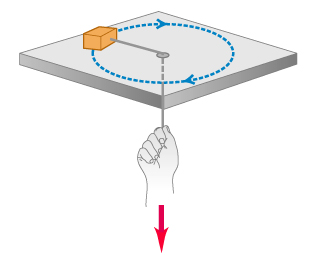# Problem: A small block with a mass of 0.0600 kg is attached to a cord passing through a hole in a frictionless, horizontal surface . The block is originally revolving at a distance of 0.41 m from the hole with a speed of 0.71 m/s . The cord is then pulled from below, shortening the radius of the circle in which the block revolves to 0.15 m . At this new distance, the speed of the block is 1.94 m/s .What is the tension in the cord in the original situation when the block has speed exttip{v_{ m 0}}{v_0} = 0.71 m/s ?What is the tension in the cord in the final situation when the block has speed exttip{v_{ m 1}}{v_1} = 1.94 m/s ?How much work was done by the person who pulled on the cord?

###### FREE Expert Solution
91% (146 ratings)
###### Problem Details

A small block with a mass of 0.0600 kg is attached to a cord passing through a hole in a frictionless, horizontal surface. The block is originally revolving at a distance of 0.41 m from the hole with a speed of 0.71 m/s . The cord is then pulled from below, shortening the radius of the circle in which the block revolves to 0.15 m . At this new distance, the speed of the block is 1.94 m/s .

What is the tension in the cord in the original situation when the block has speed = 0.71 m/s ?

What is the tension in the cord in the final situation when the block has speed = 1.94 m/s ?

How much work was done by the person who pulled on the cord?

Frequently Asked Questions

What scientific concept do you need to know in order to solve this problem?

Our tutors have indicated that to solve this problem you will need to apply the Net Work & Kinetic Energy concept. You can view video lessons to learn Net Work & Kinetic Energy. Or if you need more Net Work & Kinetic Energy practice, you can also practice Net Work & Kinetic Energy practice problems.